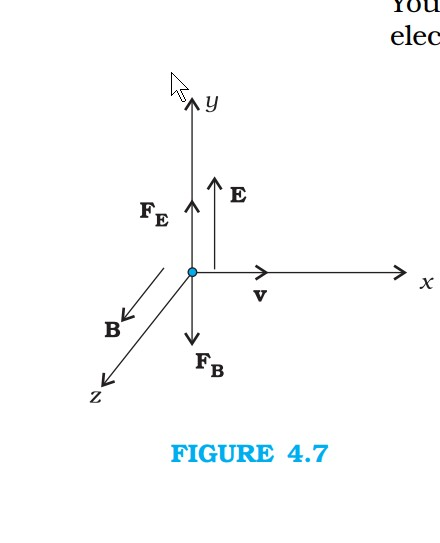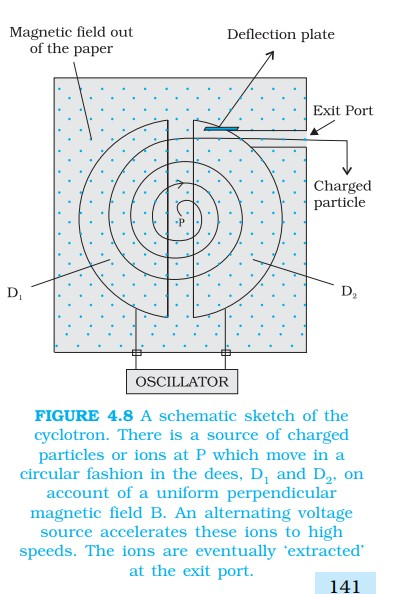Physics MOTION IN A MAGNETIC FIELD, MOTION IN COMBINED ELECTRIC AND MAGNETIC FIELDS FOR CBSE-NCERT 2

### Topic covered

color{blue}{star} MOTION IN A MAGNETIC FIELD
color{blue}{star} MOTION IN COMBINED ELECTRIC AND MAGNETIC FIELDS

### MOTION IN A MAGNETIC FIELDcolor{blue} ✍️ In the case of motion of a charge in a magnetic field, the magnetic force is perpendicular to the velocity of the particle.

color{blue} ✍️ So no work is done and no change in the magnitude of the velocity is produced (though the direction of momentum may be changed). [Notice that this is unlike the force due to an electric field, qE, which can have a component parallel (or antiparallel) to motion and thus can transfer energy in addition to momentum.]

color{blue} ✍️ We shall consider motion of a charged particle in a uniform magnetic field. First consider the case of v perpendicular to B.

color{blue} ✍️ The perpendicular force, q v × B, acts as a centripetal force and produces a circular motion perpendicular to the magnetic field.

color {blue}{➢➢} The particle will describe a circle if v and B are perpendicular to each other (Fig. 4.5).

color{blue} ✍️ If velocity has a component along B, this component remains unchanged as the motion along the magnetic field will not be affected by the magnetic field.

color{blue} ✍️ The motion in a plane perpendicular to B is as before a circular one, thereby producing a helical motion (Fig. 4.6). As we know, if r is the radius of the circular path of a particle, then a force of (m v^2) / r, acts perpendicular to the path towards the centre of the circle, and is called the centripetal force.color{blue} ✍️ If the velocity v is perpendicular to the magnetic field B, the magnetic force is perpendicular to both v and B and acts like a centripetal force. It has a magnitude q v B. Equating the two expressions for centripetal force,

color{navy}((mv^2)/r = q v B),

color {blue}{➢➢}which gives

color{navy}(r = (m v )/ (qB))

.......(4.5)

color {blue}{➢➢} for the radius of the circle described by the charged particle. The larger the momentum, the larger is the radius and bigger the circle described. If ω is the angular frequency, then color{navy}(v = ω r.) So,

color{navy}(ω = 2π ν = (q B)/ m)

...........[4.6(a)]

color {blue}{➢➢} which is independent of the velocity or energy .

color {blue}{➢➢} Here ν is the frequency of rotation. The independence of ν from energy has important application in the design of a cyclotron (see Section 4.4.2).

color {blue}{➢➢} The time taken for one revolution is color{navy}(T= (2π)/ω ≡ 1/ν.)

color{blue} ✍️ If there is a component of the velocity parallel to the magnetic field (denoted by v_(||)), it will make the particle move along the field and the path of the particle would be a helical one (Fig. 4.6).

color{blue} ✍️ The distance moved along the magnetic field in one rotation is called pitch p. Using Eq. [4.6 (a)], we have

color{navy}(p = v_(||)T = (2πm v_(||)) / q B)

..........[4.6(b)]

color {blue}{➢➢} The radius of the circular component of motion is called the radius of the helix.
Q 3135501462What is the radius of the path of an electron (mass 9 × 10^(-31) kg and charge 1.6 × 10^(–19) C) moving at a speed of 3 ×10^7 m//s in
a magnetic field of 6 × 10^(–4) T perpendicular to it? What is its frequency? Calculate its energy in keV. ( 1 eV = 1.6 × 10^(–19) J).
Class 12 Chapter example 3Solution:

Using Eq. (4.5) we find
r = m v // (qB) = 9 ×10^(–31) kg × 3 × 107 m s^(–1) // ( 1.6 × 10^(–19) C × 6 × 10^(–4) T )
26 × 10^(–2) m = 26 cm
ν = v // (2 πr) = 2×10^6 s^(–1) = 2×10^6 Hz =2 MHz.
E = (½ )mv^2 = (½ ) 9 × 10^(–31) kg × 9 × 10^(14) m^2/s^2 = 40.5 ×10^(–17) J
≈ 4×10^(–16) J = 2.5 keV.

### MOTION IN COMBINED ELECTRIC AND MAGNETIC FIELDScolor{brown}bbul("Velocity selector")
color {blue}{➢➢} You know that a charge q moving with velocity v in presence of both electric and magnetic fields experiences a force given by Eq. (4.3), that is,

color{navy}(F = q (E + v × B) = F_E +F_B)

color{blue} ✍️ We shall consider the simple case in which electric and magnetic fields are perpendicular to each other and also perpendicular to the velocity of the particle, as shown in Fig. 4.7. We have,

color{navy}(E = E hatj , " " B = B hat k ; " " v = v hat i)

color{navy}(F_E = qE = qE hatj ," " F_B = qv xx B, = q (u hat i xx B hat K) = - qB hatj)

color {blue}{➢➢} Therefore, color{brown}(F = q (E – vB)hatj .)

color{blue} ✍️ Thus, electric and magnetic forces are in opposite directions as shown in the figure. Suppose, we adjust the value of E and B such that magnitude of the two forces are equal. Then, total force on the charge is zero and the charge will move in the fields undeflected.

color {blue}{➢➢}This happens when,

color{navy}(qE= qvB) or color{navy}(v = E/B)

...............(4.7)

color{blue} ✍️ This condition can be used to select charged particles of a particular velocity out of a beam containing charges moving with different speeds (irrespective of their charge and mass).

color{blue} ✍️ The crossed E and B fields, therefore, serve as a velocity selector. Only particles with speed E/B pass undeflected through the region of crossed fields. This method was employed by J. J. Thomson in 1897 to measure the charge to mass ratio (e/m) of an electron. The principle is also employed in Mass Spectrometer – a device that separates charged particles, usually ions, according to their charge to mass ratio.

### Cyclotroncolor{blue} ✍️ The cyclotron is a machine to accelerate charged particles or ions to high energies. I

color{blue} ✍️ The cyclotron uses both electric and magnetic fields in combination to increase the energy of charged particles.

color {blue}{➢➢} As the fields are perpendicular to each other they are called crossed fields. Cyclotron uses the fact that the frequency of revolution of the charged particle in a magnetic field is independent of its energy.

color{blue} ✍️ The particles move most of the time inside two semicircular disc-like metal containers, D_1 and D_2, which are called dees as they look like the letter D.

color {blue}{➢➢} Figure 4.8 shows a schematic view of the cyclotron.

color {blue}{➢➢} Inside the metal boxes the particle is shielded and is not acted on by the electric field.

color{blue} ✍️ The magnetic field, however, acts on the particle and makes it go round in a circular path inside a dee.

color{blue} ✍️ Every time the particle moves from one dee to another it is acted upon by the electric field. The sign of the electric field is changed alternately in tune with the circular motion of the particle.

color{blue} ✍️ This ensures that the particle is always accelerated by the electric field. Each time the acceleration increases the energy of the particle. As energy increases, the radius of the circular path increases.

color {blue}{➢➢} So the path is a spiral one. The whole assembly is evacuated to minimise collisions between the ions and the air molecules. A high frequency alternating voltage is applied to the dees.

color{blue} ✍️ In the sketch shown in Fig. 4.8, positive ions or positively charged particles (e.g., protons) are released at the centre P.

color {blue}{➢➢} They move in a semi-circular path in one of the dees and arrive in the gap between the dees in a time interval T/2; where T, the period of revolution, is given by Eq. (4.6),

color{navy}(T = 1/(ν_c) = (2pim)/(qB))

color {blue}{➢➢}or color{navy}(ν_c = (qB)/(2pim))

...........(4.8)

color {blue}{➢➢} This frequency is called the cyclotron frequency for obvious reasons and is denoted by ν_c . The frequency ν_a of the applied voltage is adjusted so that the polarity of the dees is reversed in the same time that it takes the ions to complete one half of the revolution.

color {blue}{➢➢} The requirement ν_a = ν_c is called the resonance condition. The phase of the supply is adjusted so that when the positive ions arrive at the edge of D_1, D_2 is at a lower potential and the ions are accelerated across the gap.

color {blue}{➢➢} Inside the dees the particles travel in a region free of the electric field. The increase in their kinetic energy is qV each time they cross from one dee to another ( V refers to the voltage across the dees at that time). From Eq. (4.5), it is clear that the radius of their path goes on increasing each time their kinetic energy increases.

color {blue}{➢➢} The ions are repeatedly accelerated across the dees until they have the required energy to have a radius approximately that of the dees. They are then deflected by a magnetic field and leave the system via an exit slit. From Eq. (4.5) we have,

color{navy}(v = (qBR)/m)

............(4.9)

color {blue}{➢➢} where R is the radius of the trajectory at exit, and equals the radius of a dee.

color {blue}{➢➢} Hence, the kinetic energy of the ions is,

color{navy}(1/2 mv^2 = (q^2B^2R^2)/(2m))

...............(4.10)

color{blue} ✍️ The operation of the cyclotron is based on the fact that the time for one revolution of an ion is independent of its speed or radius of its orbit.

color {blue}{➢➢} The cyclotron is used to bombard nuclei with energetic particles, so accelerated by it, and study the resulting nuclear reactions.

color {blue}{➢➢} It is also used to implant ions into solids and modify their properties or even synthesis new materials. It is used in hospitals to produce radioactive substances which can be used in diagnosis and treatment.
Q 3145501463A cyclotron’s oscillator frequency is 10 MHz. What should be the operating magnetic field for accelerating protons? If the radius of its ‘dees’ is 60 cm, what is the kinetic energy (in MeV) of the proton beam produced by the accelerator.

(e =1.60 × 10^(–19) C, mp = 1.67 × 10^(–27) kg, 1 MeV = 1.6 × 10^(–13) J).
Class 12 Chapter example 4Solution:

The oscillator frequency should be same as proton’s cyclotron frequency.
Using Eqs. (4.5) and [4.6(a)] we have

B = 2π m ν//q =6.3 ×1.67 × 10^(–27) × 10^7 // (1.6 × 10^(–19)) = 0.66 T
Final velocity of protons is

v = r × 2π ν = 0.6 m × 6.3 ×10^7 = 3.78 × 10^7 m//s.

E = ½ mv^2 = 1.67 ×10^(–27) × 14.3 × 10^(14) // (2 × 1.6 × 10^(–13)) = 7 MeV.